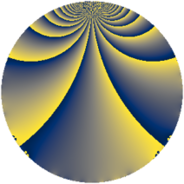# Properties

 Label 385.2.tLevel $385$ Weight $2$ Character orbit 385.t Rep. character $\chi_{385}(144,\cdot)$ Character field $\Q(\zeta_{6})$ Dimension $80$ Newform subspaces $3$ Sturm bound $96$ Trace bound $1$

# Related objects

## Defining parameters

 Level: $$N$$ $$=$$ $$385 = 5 \cdot 7 \cdot 11$$ Weight: $$k$$ $$=$$ $$2$$ Character orbit: $$[\chi]$$ $$=$$ 385.t (of order $$6$$ and degree $$2$$) Character conductor: $$\operatorname{cond}(\chi)$$ $$=$$ $$35$$ Character field: $$\Q(\zeta_{6})$$ Newform subspaces: $$3$$ Sturm bound: $$96$$ Trace bound: $$1$$ Distinguishing $$T_p$$: $$2$$

## Dimensions

The following table gives the dimensions of various subspaces of $$M_{2}(385, [\chi])$$.

Total New Old
Modular forms 104 80 24
Cusp forms 88 80 8
Eisenstein series 16 0 16

## Trace form

 $$80q + 40q^{4} - 8q^{6} + 36q^{9} + O(q^{10})$$ $$80q + 40q^{4} - 8q^{6} + 36q^{9} - 6q^{10} - 28q^{14} - 28q^{15} - 36q^{16} - 8q^{19} - 24q^{20} - 12q^{24} + 4q^{25} + 16q^{26} + 48q^{29} - 16q^{30} + 12q^{31} - 24q^{34} + 14q^{35} - 24q^{36} - 24q^{39} + 64q^{40} + 8q^{41} - 4q^{44} + 6q^{45} - 28q^{46} + 24q^{49} - 16q^{50} - 24q^{51} - 4q^{54} + 48q^{56} - 44q^{59} - 36q^{60} + 20q^{61} - 40q^{64} - 18q^{65} + 16q^{66} - 32q^{69} + 6q^{70} + 16q^{71} - 24q^{74} - 12q^{75} + 56q^{76} - 32q^{79} - 50q^{80} + 48q^{81} - 156q^{84} - 24q^{85} + 76q^{86} - 84q^{89} - 188q^{90} + 80q^{91} + 56q^{94} - 16q^{95} - 84q^{96} + O(q^{100})$$

## Decomposition of $$S_{2}^{\mathrm{new}}(385, [\chi])$$ into newform subspaces

Label Dim. $$A$$ Field CM Traces $q$-expansion
$$a_2$$ $$a_3$$ $$a_5$$ $$a_7$$
385.2.t.a $$4$$ $$3.074$$ $$\Q(\zeta_{12})$$ None $$0$$ $$0$$ $$-2$$ $$0$$ $$q+\zeta_{12}q^{2}+(-\zeta_{12}+\zeta_{12}^{3})q^{3}-\zeta_{12}^{2}q^{4}+\cdots$$
385.2.t.b $$36$$ $$3.074$$ None $$0$$ $$0$$ $$2$$ $$0$$
385.2.t.c $$40$$ $$3.074$$ None $$0$$ $$0$$ $$0$$ $$0$$

## Decomposition of $$S_{2}^{\mathrm{old}}(385, [\chi])$$ into lower level spaces

$$S_{2}^{\mathrm{old}}(385, [\chi]) \cong$$ $$S_{2}^{\mathrm{new}}(35, [\chi])$$$$^{\oplus 2}$$